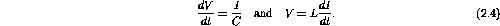Next: RC Circuit Up: Alternating Current Circuits Previous: Inductance

# Circuit Equations

Recall that voltage V is related to current I, via the passive DC circuit element resistance R, by Ohm's law V=IR. Analogously, the change in voltage and change in current are related to the current and voltage, via the passive AC circuit elements C and L, byApplying the above three equations, along with Kirchoff's loop rule, to AC circuits results in a set of differential equations. These differential equations are linear with constant coefficients and can easily be solved for Q(t), I(t), and V(t). In general the solutions will consist of a transient response and a steady-state response. The transient response describes the return to equilibrium after the EMFs change suddenly. The steady-state response describes the long term behaviour when the circuit is driven by a sinusoidal source.

We will first consider the transient response. This will be one of the few times we consider non-oscillating AC behaviour. Since Ohm's law and Kirchoff's laws are linear we can use complex exponential signals and take real or imaginary parts in the end. This is not true for power, since it is non-linear (product of signals).

Doug Gingrich
Tue Jul 13 16:55:15 EDT 1999